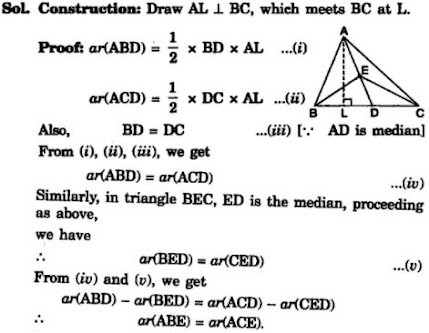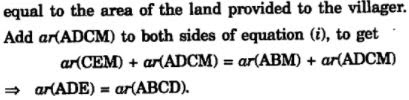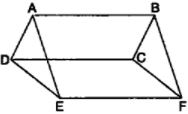# NCERT Solutions for Chapter 9 Areas of Parallelograms and Tringles Class 9 Maths

 Chapter Name NCERT Solutions for Chapter 9 Areas of Parallelograms and Triangles Class Class 9 Topics Covered Area of TrianglesArea of ParallelogramsArea between two parallel lines Related Study Materials NCERT Solutions for Class 9 MathsNCERT Solutions for Class 9Revision Notes for Chapter 9 Areas of Parallelograms and Triangles Class 9 MathsImportant Questions for Chapter 9 Areas of Parallelograms and Triangles Class 9 MathsMCQ for for Chapter 9 Areas of Parallelograms and Triangles Class 9 Maths

## Short Revision for Ch 9 Areas of Parallelograms and Triangles Class 9 Maths

1. Congruent figures have the same shape and the same size.
2. Congruent figures have equal areas.
3. Figures having equal areas may or may not be congruent.
4. If the figures have a common base and the vertices (or the vertex) opposite to the common base of each figure lie on a line parallel to the base, then the figures are said to be on the same base and between the same parallels.
5. Parallelograms on the same base (or equal bases) and between the same parallels are equal in area.
6. Triangles on the same base (or equal bases) and between the same parallels are equal in area.
7. If a triangle and a parallelogram are on the same base (or equal bases) and between the some parallels, then the area of the triangle is half of the area of the parallelogram.
8. Each median of a triangle divides it into two triangles of equal areas.
9. Area of a triangle is half the product of its base and the corresponding altitude.
10. Area of a parallelogram is the product of its base and the corresponding altitude.
11. The area of a trapezium is half of the product of sum of two parallel sides and the perpendicular distance between them.
12. The area of a rhombus is half of the product of the lengths of its diagonals.
13. The diagonals of a parallelogram divide it into four triangles of equal areas.

### Exercise 9.1

1. Which of the following figures lie on the same base and in-between the same parallels? In such a case, write the common base and the two parallels.

### Exercise 9.2

1. In Fig. 9.15, ABCD is a parallelogram, AE ⊥ DC and CF ⊥ AD. If AB = 16 cm, AE = 8 cm and CF = 10 cm, find AD.

2. If E, F, G and H are respectively the mid-points of the sides of a parallelogram ABCD, show that ar (EFGH) = 1/2 ar(ABCD).

3. P and Q are any two points lying on the sides DC and AD respectively of a parallelogram ABCD.
Show that ar(APB) = ar(BQC).

4. In Fig. 9.16, P is a point in the interior of a parallelogram ABCD. Show that
(i) ar(APB) + ar(PCD) = ½ ar(ABCD)
(ii) ar(APD) + ar(PBC) = ar(APB) + ar(PCD)
[Hint : Through P, draw a line parallel to AB.]

5. In Fig. 9.17, PQRS and ABRS are parallelograms and X is any point on side BR. Show that
(i) ar (PQRS) = ar (ABRS)
(ii) ar (AXS) = ½ ar (PQRS)

6. A farmer was having a field in the form of a parallelogram PQRS. She took any point A on RS and joined it to points P and Q. In how many parts the fields is divided? What are the shapes of these parts? The farmer wants to sow wheat and pulses in equal portions of the field separately. How should she do it?

### Exercise 9.3

1. In Fig.9.23, E is any point on median AD of a ΔABC. Show that ar (ABE) = ar(ACE).2. In a triangle ABC, E is the mid-point of median AD. Show that ar(BED) = ¼ ar(ABC).

3. Show that the diagonals of a parallelogram divide it into four triangles of equal area.

4. In Fig. 9.24, ABC and ABD are two triangles on the same base AB. If line- segment CD is bisected by AB at O, show that: ar(ABC) = ar(ABD).

5. D, E and F are respectively the mid-points of the sides BC, CA and AB of a ΔABC.
Show that
(i) BDEF is a parallelogram.
(ii) ar(DEF) = ¼ ar(ABC)
(iii) ar (BDEF) = ½ ar(ABC)

6. In Fig. 9.25, diagonals AC and BD of quadrilateral ABCD intersect at O such that OB = OD.
If AB = CD, then show that:
(i) ar (DOC) = ar (AOB)
(ii) ar (DCB) = ar (ACB)
(iii) DA || CB or ABCD is a parallelogram.
[Hint : From D and B, draw perpendiculars to AC.]

7. D and E are points on sides AB and AC respectively of ΔABC such that ar(DBC) = ar(EBC). Prove that DE || BC.

8. XY is a line parallel to side BC of a triangle ABC. If BE || AC and CF || AB meet XY at E and F respectively, show that
ar(ΔABE) = ar(ΔACF)

9. The side AB of a parallelogram ABCD is produced to any point P. A line through A and parallel to CP meets CB produced at Q and then parallelogram PBQR is completed (see Fig. 9.26). Show that
ar(ABCD) = ar(PBQR).
[Hint : Join AC and PQ. Now compare ar(ACQ) and ar(APQ).]

10. Diagonals AC and BD of a trapezium ABCD with AB || DC intersect each other at O. Prove that ar (AOD) = ar (BOC).

11. In Fig. 9.27, ABCDE is a pentagon. A line through B parallel to AC meets DC produced at F.
Show that
(i) ar(△ACB) = ar(△ACF)
(ii) ar(AEDF) = ar(ABCDE)

12. A villager Itwaari has a plot of land of the shape of a quadrilateral. The Gram Panchayat of the village decided to take over some portion of his plot from one of the corners to construct a Health Centre. Itwaari agrees to the above proposal with the condition that he should be given equal amount of land in lieu of his land adjoining his plot so as to form a triangular plot. Explain how this proposal will be implemented.13. ABCD is a trapezium with AB || DC. A line parallel to AC intersects AB at X and BC at Y. Prove that ar (△ADX) = ar (△ACY).
[Hint : Join CX.]

14. In Fig. AP || BQ || CR. Prove that ar(△AQC) = ar(△PBR).

15. Diagonals AC and BD of a quadrilateral ABCD intersect at O in such a way that ar(△AOD) = ar(△BOC). Prove that ABCD is a trapezium.

16. In Fig., ar(DRC) = ar(DPC) and ar(BDP) = ar(ARC). Show that both the quadrilaterals ABCD and DCPR are trapeziums.

Solution

### Exercise 9.4(Optional)

1. Parallelogram ABCD and rectangle ABEF are on the same base AB and have equal areas. Show that the perimeter of the parallelogram is greater than that of the rectangle.

2. In Fig. , D and E are two points on BC such that BD = DE = EC.
Show that ar (ABD) = ar (ADE) = ar (AEC).
Can you now answer the question that you have left in the ‘Introduction’ of this chapter, whether
the field of Budhia has been actually divided into three parts of equal area?
[Remark: Note that by taking BD = DE = EC, the triangle ABC is divided into three triangles ABD, ADE and AEC of equal areas. In the same way, by dividing BC into equal parts and joining the points of division so obtained to the opposite vertex of BC, you can divide DABC into triangles of equal areas.]

3. In Fig.  ABCD, DCFE and ABFE are parallelograms. Show that ar (ADE) = ar (BCF).4. In Fig. 9.32, ABCD is a parallelogram and BC is produced to a point Q such that AD = CQ. If AQ intersect DC at P, show that ar (BPC) = ar (DPQ).
[Hint : Join AC.]

5. In Fig.9.33, ABC and BDE are two equilateral triangles such that D is the mid-point of BC. If AE intersects BC at F, show that:
(i) ar (BDE) =1/4 ar (ABC)
(ii) ar (BDE) = ½ ar (BAE)
(iii) ar (ABC) = 2 ar (BEC)
(iv) ar (BFE) = ar (AFD)
(v) ar (BFE) = 2 ar (FED)
(vi) ar (FED) = 1/8 ar (AFC)

6. Diagonals AC and BD of a quadrilateral ABCD intersect each other at P. Show that
ar (APB)×ar (CPD) = ar (APD)×ar (BPC).
[Hint : From A and C, draw perpendiculars to BD.]

7. P and Q are respectively the mid-points of sides AB and BC of a triangle ABC and R is the mid-point of AP, show that:
(i) ar (PRQ) = ½ ar (ARC)
(ii) ar (RQC) = (3/8) ar (ABC)
(iii) ar (PBQ) = ar (ARC)

8. In Fig. 9.34, ABC is a right triangle right angled at A. BCED, ACFG and ABMN are squares on the sides BC, CA and AB respectively. Line segment AX ^ DE meets BC at Y. Show that:

(i) ΔMBC ≅ ΔABD
(ii) ar(BYXD) = 2ar(MBC)
(iii) ar(BYXD) = ar(ABMN)
(iv) ΔFCB ≅ ΔACE
(v) ar(CYXE) = 2ar(FCB)
(vi) ar(CYXE) = ar(ACFG)
(vii) ar(BCED) = ar(ABMN)+ar(ACFG)
Note : Result (vii) is the famous Theorem of Pythagoras. You shall learn a simpler proof of this theorem in Class X.